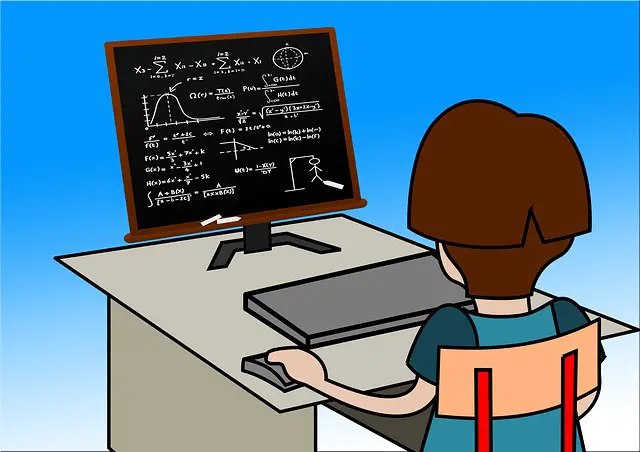# 79 Challenging Physics Problems and Questions-Physics Question Bank

Last updated on February 12th, 2022 at 02:16 pm

Here we are publishing a large number of physics problems and questions, with selective hints as well.

These numerical problems and questions are distributed among 7 sets of Questions for you.

The first set (Set number 1) is being published on this post body itself and the rest of the sets (6 more) are being embedded in a pdf file, which is also available right on this page for you.

The Standard of these sets is MEDIUM (not so hard). Please check and attempt these. We are coming up with more numerical in the coming days, which will have harder sets as well.

## Physics Problems and Questions: Question Bank-Set Number 1

Physics Problems and Questions for School and Competitive Examinations and Tests

1) A stone is falling freely from rest and the total distance covered by it in the last second of its motion equals the distance covered by it in the first 3 seconds of its motion. How long does the stone remain in the air?

2) A circular track of radius 100 m is banked at an angle of 30°. If the coefficient of friction between the wheels of a car and the road is 0.5, then what is the (i) optimum speed of the car to avoid wear and tear on its tires, and (ii) maximum permissible speed to avoid slipping?

3) The velocity of an object is V1=8 m/s towards N and the velocity of a different object is V2= 6 m/s towards West. Determine V1-V2.

4) A 20 kg iron block is kept on a horizontal table. It is pulled with a rope which is at 30 degrees with the horizontal floor. If the coefficient of friction is 0.4 then what is the minimum force required along the rope to pull the block?

5) What is the value of the coefficient of static friction of an inclined plane where a mass of 50 kg is tending to slip downwards when the angle of inclination is made 15 degrees?

6) What is the mechanical advantage?

7) A force of 200 N makes an angle of theta with the X-axis and has a scalar Y component of 30 N.
Find both the scalar X component of the force and angle theta.

8) Write and derive the work-energy theorem.

9) A 50 kg bucket is being lifted by a rope. The rope will not break if the tension is 525 N or less. The bucket started at rest and after being lifted 3 meters, it’s moving at 5 m/s. If the acceleration is constant, is the rope in danger of breaking?

10) Two blocks of 4 kg and 5 kg are in contact on a frictionless table. If a force of 3 N is applied on 2 kg block, then what will be the force of contact between the 2 blocks?

11) Can we have the value of the coefficient of friction more than one?

12) Define the equilibrium of a system?

13) An object of mass M kg moving with a velocity of 20 m/s is stopped in 2 secs by applying a force against its motion. What is the magnitude of the force?

14) Can you deduce the first law of Newtonian motion from the 2nd law?

15) ‘Pulling’ a box or ‘pushing’ it on the horizontal floor. Which one is easier and why (show with FBD / Vector)

## Question Bank-Set Number 2-7 (Physics Problems and Questions)

As said earlier, 6 more sets with awesome numerical problems and questions are here for you, within the following embedded pdf.

How to use the viewer?
-Just scroll or flip with the tools given
-There is a full-screen option (at top mid)
and then solve the challenges.

If you want links to good online materials on fundamental physics, then please subscribe to the newsletter by filling up the ‘subscription form’, it is not going to take even a minute.
Remember to validate your email ID in this process.

Physics-Question-Bank-7-sets_V2